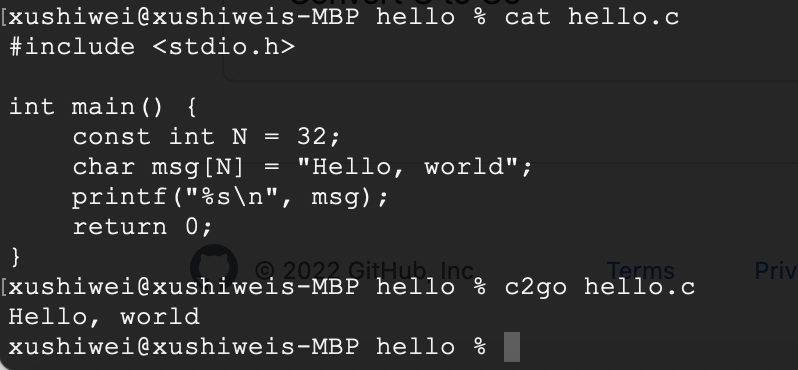# c2go – Convert C to Go

This is a subproject of the Go+ project. Its goal is converting any C project into Go without any human intervention and keeping performance close to C.## How to run examples?

Run an example:

• Build c2go tools: `go install -v ./...`
• Go `testdata/xxx` directory, and run `c2go .`

Run/Test multiple examples:

• Run examples: `c2go ./...`
• Test examples: `c2go -test ./...`

## How c2go is used in Go+

```import "C"

C.printf C"Hello, c2go!\n"
C.fprintf C.stderr, C"Hi, %7.1f\n", 3.14```

Here we use `import "C"` to import libc. It’s an abbreviation for `import "C/github.com/goplus/libc"`. It is equivalent to the following code:

```import "C/github.com/goplus/libc"

C.printf C"Hello, c2go!\n"
C.fprintf C.stderr, C"Hi, %7.1f\n", 3.14```

In this example we call two C standard functions `printf` and `fprintf`, passing a C variable `stderr` and two C strings in the form of `C"xxx"` (a Go+ syntax to represent C style strings).

Run `gop run .` to see the output of this example:

``````Hello, c2go!
Hi,     3.1
``````

## What’s our plan?

• First, support most of the syntax of C. Stage: `Done Almost`, see supported C syntax.
• Second, compile `sqlite3` to fix c2go bugs and get list of its dependent C standard libary fuctions. Stage: `Done`, see github.com/goplus/sqlite and its dependent fuctions.
• Third, support most of C standard library functions (especially that used by `sqlite3`), and can import them by Go+. Stage: `Doing`, see detailed progress here.
• Last, support all custom libraries, especially those well-known open source libraries. Stage: `Planning`.

## Tested Platform

• MacOS: 1.17.x
• Linux: ubuntu-20.04 (temporarily skip `testdata/qsort`)
• Windows

## Supported C syntax

### Data structures

• Void: `void`
• Boolean: `_Bool`, `bool`
• Integer: [`signed`/`unsigned`] [`short`/`long`/`long long`] `int`
• Enum: `enum`
• Float: `float`, `double`, `long double`
• Character: [`signed`/`unsigned`] `char`
• Wide Character: `wchar_t`
• Large Integer: [`signed`/`unsigned`] `__int128`
• Complex: `_Complex` `float`/`double`/`long double`
• Typedef: `typedef`
• Pointer: *T, T[]
• Array: T[N], T[]
• Array Pointer: T(*)[N]
• Function Pointer: T (*)(T1, T2, …)
• Struct: `struct`
• Union: `union`
• BitField: `intType :N`

### Operators

• Arithmetic: a+b, a-b, a*b, a/b, a%b, -a, +a
• Increment/Decrement: a++, a–, ++a, –a
• Comparison: a<b, a<=b, a>b, a>=b, a==b, a!=b
• Logical: a&&b, a||b, !a
• Bitwise: a|b, a&b, a^b, ~a, a<<n, a>>n
• Pointer Arithmetic: p+n, p-n, p-q, p++, p–
• Assignment: `=`
• Operator Assignment: a`<op>=`b
• BitField Assignment: `=`
• BitField Operator Assignment: a`<op>=`b
• Struct/Union/BitField Member: a.b
• Array Member: a[n]
• Pointer Member: &a, *p, p[n], n[p], p->b
• Comma: `a,b`
• Ternary Conditional: cond?a:b
• Function Call: f(a1, a2, …)
• Conversion: (T)a
• Sizeof: sizeof(T), sizeof(a)
• Offsetof: __builtin_offsetof(T, member)

### Literals

• Boolean, Integer
• Float, Complex Imaginary
• Character, String
• Array: `(T[]){ expr1, expr2, ... }`
• Array Pointer: `&(T[]){ expr1, expr2, ... }`
• Struct: `struct T{ expr1, expr2, ... }`

### Initialization

• Basic: `T a = expr`
• Array: `T a[] = { expr1, expr2, ... }`, `T a[N] = { expr1, expr2, ... }`
• Struct: `struct T a = { expr1, expr2, ... }`, `struct T a = { .a = expr1, .b = expr2, ... }`
• Union: `union T a = { expr }, union T a = { .a = expr }`
• Array in Struct: `struct { T a[N]; ... } v = { { expr1, expr2, ... }, ... }`, `struct { T a[N]; ... } v = { { .a = expr1, .a = expr2, ... }, ... }`

### Control structures

• If: `if (cond) stmt1 [else stmt2]`
• Switch: `switch (tag) { case expr1: stmt1 case expr2: stmt2 default: stmtN }`
• For: `for (init; cond; post) stmt`
• While: `while (cond) stmt`
• Do While: `do stmt while (cond)`
• Break/Continue: `break`, `continue`
• Goto: `goto label`

• Parameters